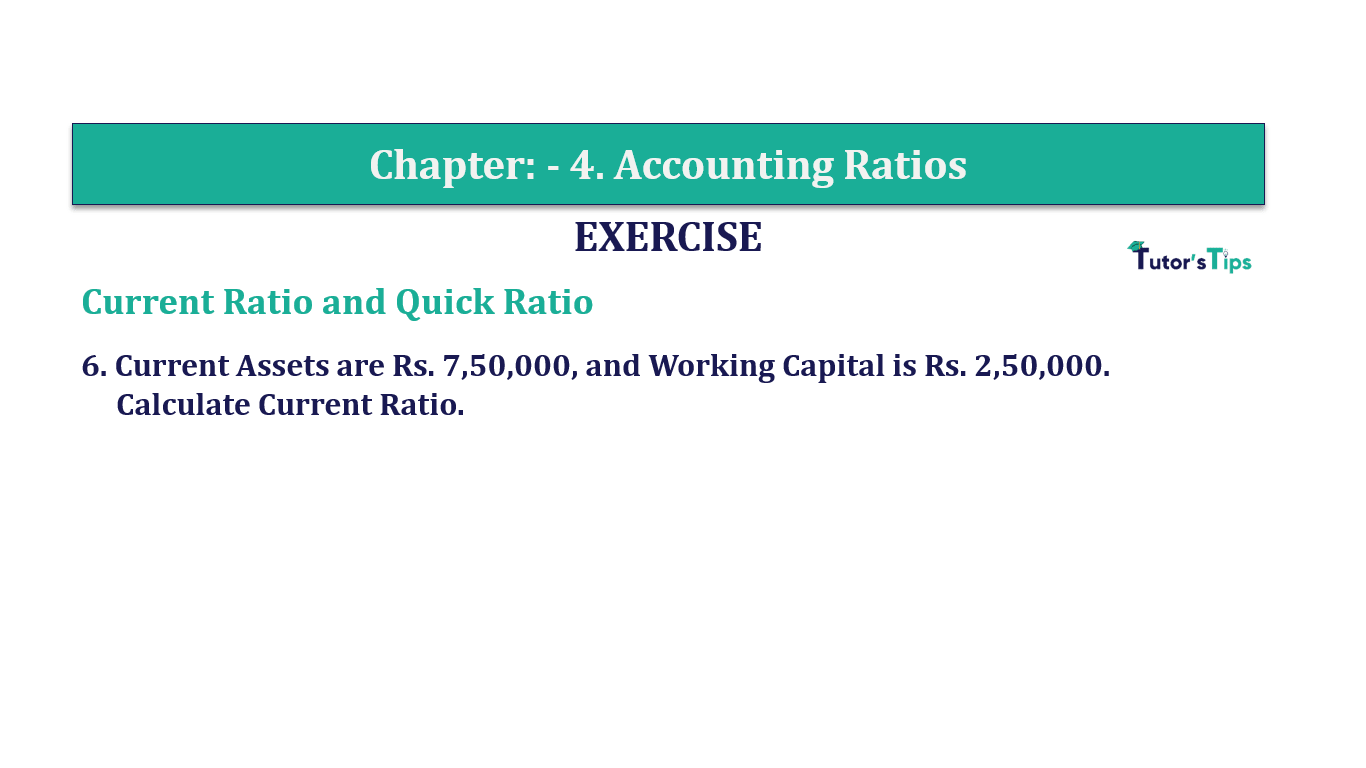# Question 6 Chapter 4 of +2-B – T.S. Grewal 12 ClassQuestion No. 6 - Chapter No.4 - T.S. Grewal +2 Book Part B

Question 6 Chapter 4 of +2-B

Current Ratio and Quick Ratio

6. Current Assets are Rs. 7,50,000, and Working Capital is Rs. 2,50,000.
Calculate Current Ratio.

### The solution of Question 6 Chapter 4 of +2-B: –

 Current Assets =Rs. 7,50,000
 Working Capital= Rs. 2,50,000
 Working Capital= Current Assets – Current Liabilities
 Rs. 7,50,000 – Current Liabilities
 Rs. 7,50,000 – Rs. 2,50,000
 Current Liabilities = Rs. 5,00,000

 Current Ratio= Current Assets Current Liabilities
 Current Ratio= 7,50,000 5,00,000

Current Ratio=1.5 : 1

Balance Sheet: Meaning, Format & Examples

Comment if you have any question.

Also, Check out the solved question of previous Chapters: –